## Find the missing side or angle. Round to the nearest tenth. A=15° C= 120° b=3 c=[? ] Enter

Question

Find the missing side or angle.
Round to the nearest tenth.
A=15°
C= 120°
b=3
c=[? ]
Enter

in progress 0
6 months 2021-07-25T05:27:52+00:00 1 Answers 12 views 0

1. Given: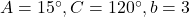.

To find:

The length of side c.

Solution:

According to the angle sum of property of a triangle, the sum of all interior angles of a triangle is 180 degrees.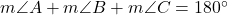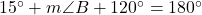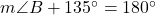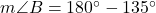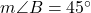According to the Law of sines,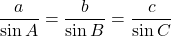Using Law of sines, we get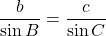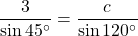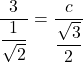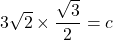On further simplification, we get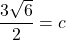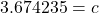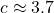Therefore, the length of side c is about 3.7 units.